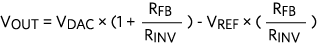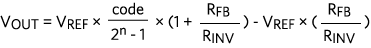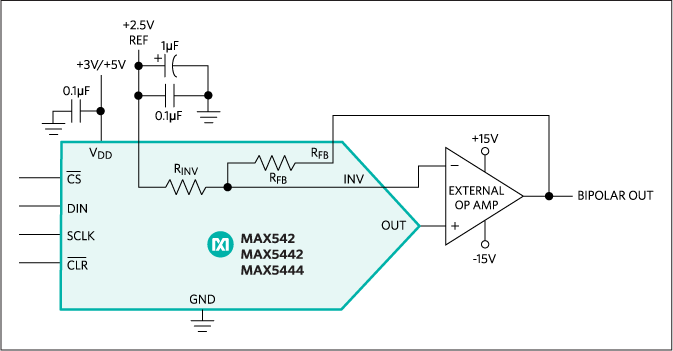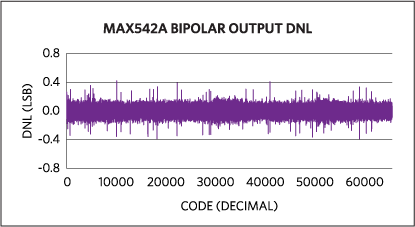# Challenge the Conventional - Make Unipolar DACs Bipolar

### Abstract

This application note investigates how to convert a unipolar digital-to-analog converter (DAC) for use in bipolar applications. Calculations are provided to show the conversion for an ideal unipolar DAC through the addition of a precision voltage reference and a precision op amp. Real-world conversion is illustrated with discussions on how to choose needed resistors, a voltage reference, and an op amp as well as the parameters to consider.

A similar version of this article was published March 3, 2014 in EE Herald.

#### Introduction

The trend in analog ICs is toward single-supply digital-to-analog converters (DACs). A DAC with only a positive 5V supply is convenient, but it limits the available applications to those not requiring high-voltage, high-current, or bipolar (±) outputs. In this application note, we illustrate how an external operational amplifier can transform a unipolar DAC to provide bipolar operation.

#### Take the Unconventional Route

The expression “up the down staircase” comes from a movie, a play, and a book by the same name.1 It is a comedy based on a school in New York City. The title recalls a rule that penalized students for going up the staircase reserved for coming down. It is always a great temptation for a youngster to run up stairs, or escalators (Figure 1), that are going down. Some might say that the child is “thinking outside the box” or breaking the rules, and perhaps he is. Clearly, he is challenging the expected or mandated flow, the common thinking. He also shows how with some daring, a goal can be attained by an unconventional route. There is a lesson here for us engineers.Figure 1. The boy running up this escalator is using an unconventional way to reach his goal, probably the toy section. We engineers can take note and try to solve problems with unconventional methods.

Sometimes when we design analog circuits, the design “elements” just do not want to fit together. The solution seems unusually elusive. An example situation is when we need a bipolar output from a unipolar DAC. The industry’s trend today is toward smaller, lower power, and higher performance devices, which is excellent when that resolves a solution problem. However, this same low-voltage, unipolar DAC cannot function directly in high-performance, high-voltage, high-current, or bipolar applications. Any additional circuits must not degrade the DAC’s performance. In such a case, it is time to go up that escalator, to try something different. We show you how to make a bipolar output from a unipolar DAC by adding a high-voltage op amp.

#### Modifying an “Ideal” Unipolar DAC

A simple bipolar output circuit is shown in Figure 2. It contains a unipolar DAC, a precision voltage reference, and a precision op amp.Figure 2. Typical bipolar output operating circuit.

The output functionality of this circuit can be derived by making two common assumptions about an ideal op amp:

• The input op amp current is 0.
• The V+ input is equal to the V- input at stable conditions.

Following Kirchhoff’s current law, the equation for the V- node is:(Eq. 1)

Solving Equation 1 for VOUT and replacing the V- with VDAC:(Eq. 2)

In fact, we have derived the equation of a differential amplifier where the first element is the noninverting input and the second element is the inverting component, each with their gains.

Since the DAC output, VDAC, is a function of the input code and the supplied reference voltage, Equation 2 can be rewritten as:(Eq. 3)

If RFB = RINV and their ratio becomes 1, this equation can be further simplified to:(Eq. 4)

Thus, bipolar operation allows the output to swing from -VREF to +VREF with the unity gain. Table 1 shows the ideal bipolar output data versus code based on Equation 4, for the ideal 16-bit DAC with 2.5V VREF in Figure 2.

Table 1. Bipolar Output versus Code (VREF = 2.5V)

Decimal Code Binary Code Hex Code VOUT (V)
0 0000 0000 0000 0000 0 -2.50000000
1 0000 0000 0000 0001 1 -2.49992370
2 0000 0000 0000 0010 2 -2.49984741
3 0000 0000 0000 0011 3 -2.49977111
4 0000 0000 0000 0100 4 -2.49969482
5 0000 0000 0000 0101 5 -2.49961852
6 0000 0000 0000 0110 6 -2.49954223
7 0000 0000 0000 0111 7 -2.49946593
8 0000 0000 0000 1000 8 -2.49938964
9 0000 0000 0000 1001 9 -2.49931334
10 0000 0000 0000 1010 A -2.49923705
11 0000 0000 0000 1011 B -2.49916075
12 0000 0000 0000 1100 C -2.49908446
13 0000 0000 0000 1101 D -2.49900816
14 0000 0000 0000 1110 E -2.49893187
15 0000 0000 0000 1111 F -2.49885557
. . . .
. . . .
. . . .
32767 0111 1111 1111 1111 7FFF -0.00003815
32768 1000 0000 0000 0000 8000 0.00003815
32769 1000 0000 0000 0001 8001 0.00011444
. . . .
. . . .
. . . .
65534 1111 1111 1111 1110 FFFE 2.49992370
65535 1111 1111 1111 1111 FFFF 2.50000000

#### Optimizing the “Ideal” DAC for a Real-World Application

As we see, it was easy to convert our ideal unipolar DAC. However, we are living in the real world where nothing is ideal. Each component in Figure 2 brings its own level of accuracy, which collectively contributes to the DAC’s final output accuracy. Each system must be characterized and calibrated to the accuracy required by the application. As a result, even though you might select a high-precision 16-bit DAC, special attention should also be paid to selecting the appropriate voltage reference, amplifier, and feedback resistors. Which component contributes the most to inaccuracy? Which parameters are most critical for bipolar applications? These are neither simple, nor trivial questions. An inexperienced engineer might be surprised to learn that even simple resistors can be very critical to this design modification.

#### Selecting the Right Resistors Is Not Simple

Resistance matching, tolerance, and temperature coefficient are the most important parameters in any precision application. These parameters contribute to circuit errors, offset, gain error, and gain stability over the temperature range. Each parameter needs to be considered.

There is a wide range of resistor types available, from thin film to metal foil and with tolerance from 1% down to 0.01%. Temperature coefficients range from 300ppm/°C to 0.2ppm/°C with costs that track the precision. However, the most important parameter for setting gain might not be cited explicitly in the resistor’s data sheet: the resistor’s matching to another resistor. For production of more than a few pieces where the resistors can be hand-matched, one must assume that two resistors are at opposite ends of the tolerance. This is the only assumption that allows safe operation in the worst-case situation. Precision-matched resistor pairs can be expensive, depending on the manufacturing process. The great advantage of using a semiconductor fabrication process is that the resistors are made in a photoduplication process and manufactured simultaneously on the same substrate. There are two ways to accomplish this. One method uses a product with just two resistors in the package.2 The other method uses multiple resistors and a DAC that are matched in the same package. We explain this second method below.

#### Choosing the Right Amplifier

Selecting the right amplifier can also be challenging, especially for a 16-bit and higher precision DAC. Close attention must be paid to the input parameters. There are many: input bias current, input offset voltage, input offset-voltage drift, input voltage range, input capacitance and settling time, and input current and voltage noise density. Other parameters are equally important: common-mode rejection ratio (CMRR) and power-supply rejection ratio (PSRR), total harmonic distortion (THD) and gain bandwidth, slew rate, and output transient recovery time. A detailed explanation of each of these parameters lies outside the scope of this article and requires a thorough examination of the amplifier’s data sheet.3

#### External Factors Impact the Voltage Reference

There are several key specifications for choosing a voltage reference,4 and you need to consult the data sheet for each: output voltage accuracy, output voltage temperature coefficient, line and load regulation, and output voltage noise and long-term stability. After all this, there is yet another consideration. External forces can degrade some voltage reference parameters.5 For example, load regulation can become an issue if the DAC’s structure changes the load on the voltage reference.

To better understand this process, we consider three different scenarios.

1. Suppose that there is a DAC with a buffered reference input and it maintains the same impedance on the reference pin for all output levels and digital codes. However, without a reference input buffer, the application accuracy can degrade if the load changes during operation.
2. Consider now a string DAC architecture, otherwise known as a digital potentiometer, which in its simplest form is a group of series-connected resistors with a tap and switch between each resistor so any can be chosen as the wiper. If the circuit load on the pot’s wiper is very high impedance, it will have virtually no effect on the total resistance that the voltage reference would see at the top of the pot. If, however, a lower resistance load is on the wiper, the change in load resistance seen by the voltage reference can be significant. In that case, the load regulation defined in the data sheet can have a significant impact on the application accuracy.
3. There is also a rail-to-rail (R-2R) ladder. With an R-2R structure, the voltage reference load can change by 10x to 20x, and the additional load from the resistors, RINV and RFB, and the amplifier dictate that the voltage reference’s resistance should be as high as possible.

#### Making the Unconventional Practical—the Desired Bipolar DAC

Unipolar, 16-bit, unbuffered DACs can perform bipolar operation with the addition of an external precision op amp. Two examples of such a configuration are the 16-bit MAX542 and MAX5442 DACs which use integrated 0.015% (max) matched scaling resistors, RFB and RINV, for an easy bipolar output swing (Figure 3). Use of these DACs eliminates duplication of output buffers, saves PCB real estate, and provides an easy-to-use and cost-effective solution for our customers.Figure 3. These 16-bit DACs use an external op amp to provide a bipolar output.

This solution requires the latest generation of op amps, such as the MAX9632. The INL and DNL graphs of bipolar operation for the DACs in Figure 3 are shown in Figures 4 to 7. The INL calculation was made using nonadjusted data measured by an Agilent®-HP® 3458A multimeter and utilizing the end-points method.Figure 4. Bipolar output INL for the MAX542A.Figure 5. Bipolar output DNL for the MAX542A.Figure 6. Bipolar output INL for the MAX5442A.Figure 7. Bipolar output DNL for the MAX5442A.

#### Conclusion

Although not as simple in a real-world scenario, converting a unipolar DAC for use in applications that require bipolar operation is doable if you think outside the box, or about walking up the down staircase. By adding resistors, a precision voltage reference, and a precision op amp to a unipolar DAC, we succeeded in doing just that.

References

1. Up the Down Staircase (book) http://en.wikipedia.org/wiki/Up_the_Down_Staircase, (film) http://en.wikipedia.org/wiki/Up_the_Down_Staircase_%28film%29.
2. For some examples of appropriate resistors, see the MAX5490 data sheet (a 100kΩ precision-matched resistor-divider), the MAX5491 data sheet (a precision-matched resistor-divider), and the MAX5492 data sheet (a 10kΩ precision-matched resistor-divider).
3. See also tutorial 4348, “Minimize Voltage Offsets in Precision Amplifiers” and application note 4295, “Small-Signal Bandwidth in a Big-Band Era.”
4. See Maxim’s portfolio of voltage references, which includes more than 140 series precision voltage references with wide voltage ranges (1.2V to10V).
5. For more information, see tutorial 719, “Understanding Voltage-Reference Topologies and Specifications” and application note 2879, “Selecting the Optimum Voltage Reference.”